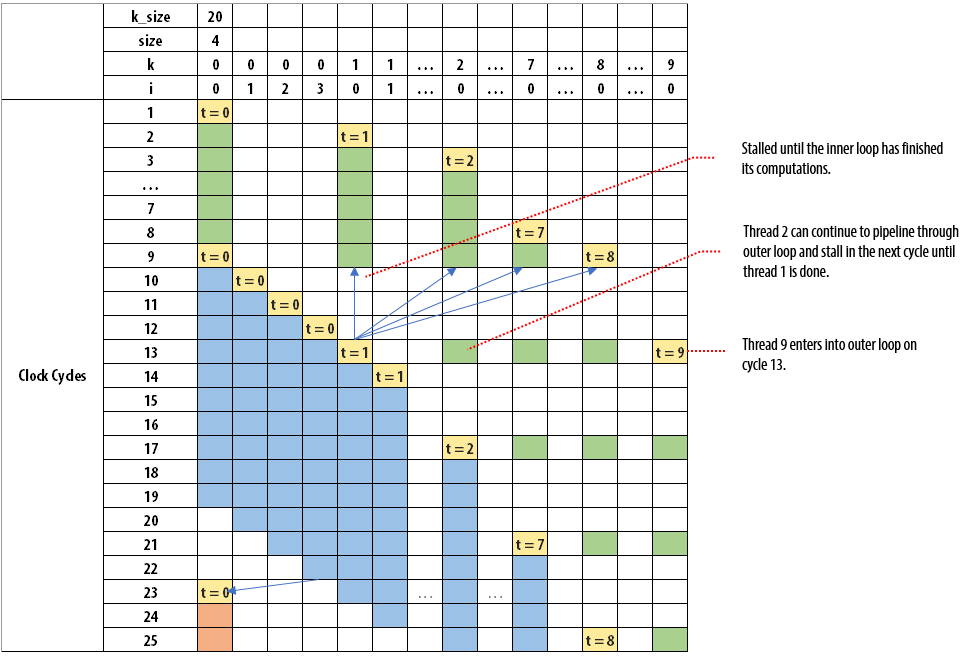ID 683521
Date 3/28/2022
Public

## 1.3. Single Work-Item Kernel versus NDRange Kernel

Intel® recommends that you structure your OpenCL kernel as a single work-item, if possible. However, if your kernel program benefits from explicitly describing multiple concurrent threads, you can structure your application as an NDRange kernel because the kernel can execute multiple work-items concurrently.

When a kernel describes a single work item, the Intel® FPGA SDK for OpenCL™ host can execute the kernel as a single work-item, which is equivalent to launching a kernel with an NDRange size of (1, 1, 1). The compiler tries to accelerate the single work item for best performance.

The OpenCL Specification version 1.0 describes this mode of operation as task parallel programming. A task refers to a kernel executed with one work-group that contains one work-item.

Generally, the host launches multiple work-items in parallel. However, this data parallel programming model is not suitable for situations where fine-grained data must be shared among parallel work-items. In these cases, you can maximize throughput by expressing your kernel as a single work-item. Unlike NDRange kernels, single work-item kernels follow a natural sequential model similar to C programming. Particularly, you do not have to partition the data across work-items.

To ensure high-throughput single work-item-based kernel execution on the FPGA, the Intel® FPGA SDK for OpenCL™ Offline Compiler must process multiple pipeline stages in parallel at any given time. This parallelism is realized by pipelining the iterations of loops.

Consider the following simple example code that shows accumulating with a single-work item:

1 kernel void accum_swg (global int* a,
global int* c,
int size,
int k_size) {
2     int sum;
3     for (int k = 0; k < k_size; ++k) {
4        for (int i = 0; i < size; ++i) {
5            int j = k * size + i;
7        }
8     }
9     for (int k = 0; k < k_size; ++k) {
10       c[k] = sum[k];
11    }
12 }
During each loop iteration, data values from the global memory a is accumulated to sum[k]. In this example, the inner loop on line 4 has an initiation interval value of 1 with a latency of 11. The outer loop also has an initiation interval value greater than or equal to 1 with a latency of 8.
Note: The launch frequency of a new loop iteration is called the initiation interval (II). II refers to the number of hardware clock cycles for which the pipeline must wait before it can process the next loop iteration. An optimally unrolled loop has an II value of 1 because one loop iteration is processed every clock cycle.
Figure 7. System View of Single-Work Item KernelThe following figure illustrates how each iteration of i enters into the block:

Figure 8. Inner Loop accum_swg.B2 Execution

When you observe the outer loop, having an II value of 1 also means that each iteration of the thread can enter at every clock cycle. In the example, k_size of 20 and size of 4 is considered. This is true for the first eight clock cycles as outer loop iterations 0 to 7 can enter without any downstream stalling it. Once thread 0 enters into the inner loop, it takes four iterations to finish. Threads 1 to 8 cannot enter into the inner loop and they are stalled for four cycles by thread 0. Thread 1 enters into the inner loop after thread 0's iterations are completed. As a result, thread 9 enters into the outer loop on clock cycle 13. Threads 9 to 20 enters into the loop at every four clock cycles, which is the value of size. Through this example, you can observe that the dynamic initiation interval of the outer loop is greater than the statically predicted initiation interval of 1 and it is a function of the trip count of the inner loop.

Figure 9. Single Work-Item ExecutionImportant:
• Using any of the following functions causes your kernel to be interpreted as an NDRange:
• get_local_id()
• get_global_id()
• get_group_id()
• get_local_linear_id()
• barrier
• If the reqd_work_group_size attribute is specified to be anything other than (1, 1, 1), your kernel is interpreted as an NDRange. Otherwise, your kernel is interpreted as a single-work-item kernel.

Consider the same accumulate example written in NDRange:

kernel void accum_ndr (global int* a,
global int* c,
int size) {
int k = get_global_id(0);

int sum;
for (int i = 0; i < size; ++i) {
int j = k * size + i;
sum[k] += a[j];
}
c[k] = sum[k];
}
Figure 11. System View of the NDRange Kernel### Limitations

The OpenCL task parallel programming model does not support the notion of a barrier in single-work-item execution. Replace barriers (barrier) with memory fences (mem_fence) in your kernel.